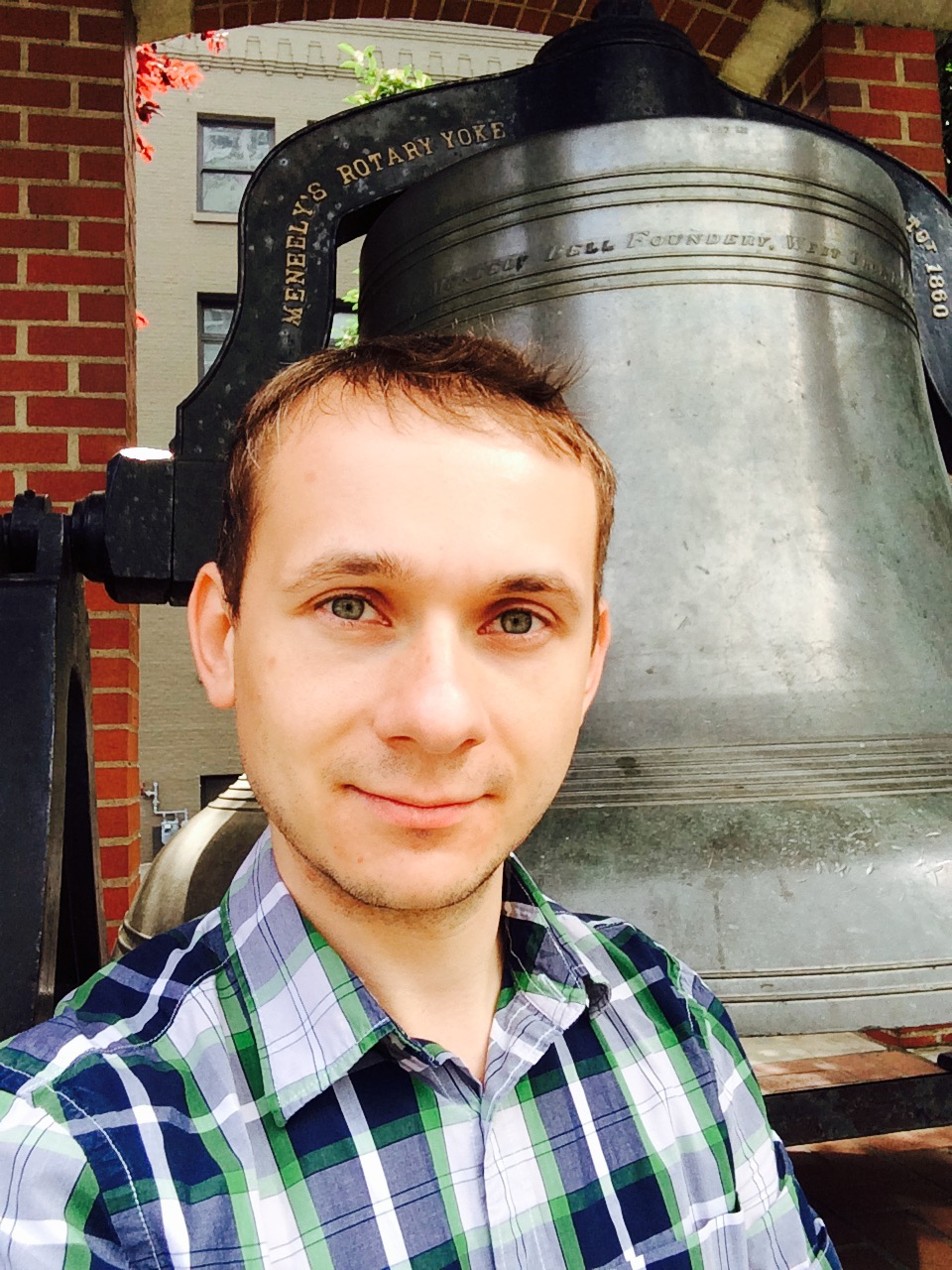Mihai Fulger Work address: Department of Mathematics University of Connecticut 341 Mansfield Road, Unit 1009 Storrs, CT 06269 USA Contact: Office: MONT 323 E-mail: mihai.fulger[AT no spam]uconn.edu Curriculum Vitae

My area of research is algebraic geometry. I am interested in questions about positivity.

Teaching Attending
MATH 5020 Topics in Algebra: Commutative Algebra Algebra seminar

Papers

1. (with T. Murayama) Seshadri constants for vector bundles (2019), submitted
2. Seshadri constants for curve classes (2017), IMRN, to appear
3. Positive cones of numerical cycle classes, Bulletin Mathematique de la Societe des Sciences Mathematiques de Roumanie, Volume 60(108)/2017, Issue no. 4, pages 399-415, in collection: honorary volume dedicated to Dorin Popescu on the occasion of his 70th birthday
4. (with B. Lehmann), Zariski decompositions of numerical cycle classes J. Algebraic Geom. (2017)
5. (with B. Lehmann), Positive cones of dual cycle classes Algebr. Geom. (2017)
6. (with B. Lehmann), Kernels of numerical pushforwards Adv. Geom. (2017)
7. (with J. Kollár and B. Lehmann), Volumes and Hilbert function of R-divisors Michigan Math. J. (2016)
8. (with B. Lehmann), Morphisms and faces of pseudo-effective cones Proc. Lond. Math. Soc. (2016)
9. (with D. Schmitz), Newton-Okounkov bodies and complexity functions, C. R. Math. Acad. Sci. Paris (2016)
10. (with X. Zhou), Asymptotic Schur decomposition of Veronese syzygy functors Math. Ann. (2015)
11. Local volumes on normal algebraic varieties Ann. Inst. Fourier (2013)
12. The cones of effective cycles on projective bundles over curves Math.Z. (2011)
13. (with M. Marchitan) Some splitting criteria on Hirzebruch surfaces, Bull. Math.Soc. Sci. Math. Roumaine (2011)

Some notes